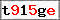This website uses cookies to improve user experience. By using our website you consent to all cookies in accordance with our Cookie Policy. X

### Basics of tile-based engine part 3: line of sight [advanced]

So we already have isometric view and path finding topic behind us, now it is time for the third and final part: field of view. Basically it usually comes down to checking whether there is a direct connection between two points (for example a player character and an enemy), without anything blocking the line. More often than not, it is usually quite a complicated issue, but luckily not in tile-based engines -we just need a line drawing algorithm. Why is that? Line drawing algorithms start at one point and move toward another one pixel by pixel, until they form a line. Now if we were to replace the drawing function with one checking the tiles, the result would be an algorithm that step by step checks a line in between two points for some kind of obstacles.
That said, we first need an algorithm - personally I recommend one from Wikipedia: Bresenham Algorithm. Implemented in ActionScript it might look like this:
```function isInSight(tiles:Vector.<Vector.<int>>,x1:Number, y1:Number, x2:Number, y2:Number):Boolean {
var dx:Number;
var dy:Number;
if (x1>x2) {
dy = y1;
y1 = y2;
y2 = dy;
dx = x1;
x1 = x2;
x2 = dx;
}
dx = x2-x1;
dy = y2 - y1;

var y:Number;
var x:Number;
var m:Number;
if (Math.abs(dx)>Math.abs(dy)) {
m = dy/dx;
y = y1;
for (x=x1; x<x2; x++) {
if(tiles[x][y] == 1) return false;
y = y+m;
}
} else {
m = dx/dy;
x = x1;
if (y1<y2) {
for (y = y1; y < y2; y++) {
if(tiles[x][y] == 1) return false;
x = x+m;
}
} else {
for (y = y1; y > y2; y--) {
if(tiles[x][y] == 1) return false;
x = x-m;
}
}
}

if(tiles[x2][y2] == 1) return false;
return true;
}
```
The isInSight function will return false when on the way from point 1 to point 2 there will be a tile marked as 1 in two-dimensional array tiles.
Easy. And the best part is that most line drawing algorithms are quite fast making this solution viable even in really big maps.

Name:
Comment:
Confirm the image code: